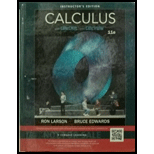Chapter 15.2, Problem 77E### Calculus (MindTap Course List)

11th Edition
Ron Larson + 1 other
ISBN: 9781337275347

#### Solutions

Chapter
Section### Calculus (MindTap Course List)

11th Edition
Ron Larson + 1 other
ISBN: 9781337275347
Textbook Problem

# Investigation The top outer edge of a solid with vertical sides and resting on the xy-plane is modeled by r ( t ) = 3 cos t i + 3 sin t j + ( 1 + sin 2 2 t ) k , where all measurements are in centimeters. The intersection of the plane y = b , where ( − 3 < b < 3 ) with the top of the solid is a horizontal line.(a) Use a computer algebra system to graph the solid.(b) Use a computer algebra system to approximate the lateral surface area of the solid.(c) Find (if possible) the volume of the solid.

(a)

To determine

To graph: The solid modeled by the function r(t)=3costi^+3sintj^+(1+sin22t)k^ by the use of a Computer algebra system

Explanation

Given:

The top outer edge of a solid with vertical sides and resting on the xy-plane is modeled by

r(t)=3costi^+3sintj^+(1+sin22t)k^ and all measurements are in centimeters. The

intersection of the plane y=b(3<b<3) with the top of the solid is a horizontal line.

Graph:

Compare r(t)=3costi^+3sintj^+(1+sin22t)k^ with the standard equation r(t)=(xt)i^+y(t)j^+z(t)k^, That is

x(t)=3cost—–(1)

y(t)=3sint——(2)

z(t)=1+sin22tz(t)=1+(sin2t)2z(t)=1+(2sintcost)2

z(t)=1+4sin2tcos2t——–(3)

The plane y=b, where 3<b<3, is constant

(b)

To determine

To calculate: The lateral surface area of the obtained solid approximately by using a CAS.

(c)

To determine

To calculate: The volume of the solid which is obtained in the above part.

### Still sussing out bartleby?

Check out a sample textbook solution.

See a sample solution

#### The Answers to Your Study Problems

Solve them all with bartleby. Boost your grades with guidance from subject experts covering thousands of textbooks. All for just \$9.99/month

Get As ASAP

## Additional Math Solutions

#### Expand each expression in Exercises 122. (2x3)(2x+3)

Finite Mathematics and Applied Calculus (MindTap Course List)

#### In problems 45-48, determine a value for that makes each statement true. 48.

Mathematical Applications for the Management, Life, and Social Sciences

#### In Exercises 110, find the graphical solution to each inequality. 3x+6y12

Finite Mathematics for the Managerial, Life, and Social Sciences